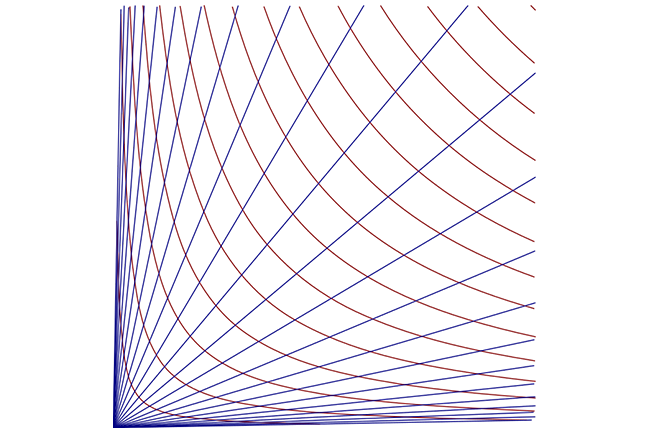## Want to keep learning?

This content is taken from the UNSW Sydney's online course, Maths for Humans: Inverse Relations and Power Laws. Join the course to learn more.
1.7

## UNSW SydneyWe can use hyperbolas as grid lines

# Hyperbolas for navigation and military use

Both the ellipse and the hyperbola have alternate descriptions in terms of sums and differences of distances to the foci. For the hyperbola, this property is quite important in radar and has navigational and military applications.

In this step we learn about

• alternative definitions of the ellipse and hyperbola

• how hyperbolas are used in the LORAN and Decca navigational systems

## The gardener’s construction of the ellipse

Around 800 AD the gardener’s construction was discovered by Arab mathematicians. Three brothers to be precise: Muhammed, Ahmad and al-Hasan Banu Musa ibn Shakir. They realised that if Muhammed and Ahmad stood in two different places holding the ends of a rope, and al-Hasan moved while keeping the rope taut, then the path al-Hasan traced out was an ellipse. The points where Muhammed and Ahmad stand are the foci of that ellipse.

Mathematically, this construction can be described by the condition that the sum of the distances from a point $$\normalsize X$$ on the ellipse to the fixed points $$\normalsize{F_1}$$ and $$\normalsize{F_2}$$ is constant. Or

$\Large{\vert F_1,X\vert + \vert X,F_2\vert=\text{constant}}.$

This is a practical method for drawing an ellipse.## The gardener’s construction of the hyperbola

There is also a corresponding description of a hyperbola as the locus of a point $$\normalsize{X}$$ such that the absolute value of the difference of the distances to the fixed points $$\normalsize{F_1}$$ and $$\normalsize{F_2}$$ is constant, that is

$\Large{\big\vert \vert F_1,X\vert - \vert X,F_2\vert \big\vert=\text{constant}}.$

These points $$\normalsize{F_1}$$ and $$\normalsize{F_2}$$ are the foci of the hyperbola.Q1 (C): Can you think of a way to draw a hyperbola using only office supplies, MacGyver?

Historically the LORAN system, developed by the USA in WWII, was a system of beacons for sea navigation which emitted radio signals at regular intervals. A similar British system called Decca was developed for use in the North Sea.Decca DeZeeuw from nl GFDL or CC-BY-SA-3.0, via Wikimedia Commons

A ship at sea could determine the difference in times between receiving two signals from given stations, allowing the navigation officers to know, using the gardener’s property of the hyperbola, that their position was on some particular hyperbola. Now if we have two such measurements, then there are two such hyperbolas that can be drawn on a map, and one of their (four) intersections will give the position of the ship. So if we add one more difference measurement, we can determine the position exactly.

Of course these days we have GPS and satellites, so this kind of location is now much simpler. But in fact the basic principle also applies to explain how GPS works.

## Locating enemy gun positions

An earlier use of the same idea of triangulating for position was developed in WWI, when soldiers wished to determine the position of enemy artillery. By measuring the exact times of the noise of a gun firing as recorded by three different observers, and determining the differences between those times, two hyperbolas could be plotted whose intersection would give the position of the enemy gun.Bundesarchiv Bundesarchiv, Bild 102-11934 / CC BY-SA 3.0 de, via Wikimedia Commons

Since opposing artillery was often many kilometres away behind enemy lines, microphones would have to be set up several hundred yards apart. Nevertheless this proved a very valuable technique for trying to locate, and hence take out, enemy guns. In fact the same idea is still very much in use these days, except that the technology is now much lighter, quicker and more effective.

So the properties of hyperbolas have been well-used for navigation and military applications!

## Discussion

Please share any thoughts you have on these topics. Are you surprised to find that conics have so many practical uses?